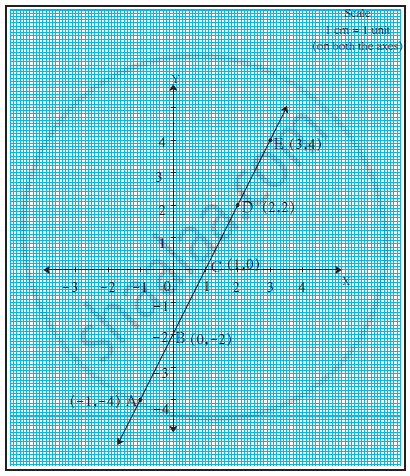Advertisement Remove all ads

# Complete the Table Below the Graph with the Help of the Following Graph. - Geometry

Complete the table below the graph with the help of the following graph.Sr. No. First point Second point Co-ordinates of first point (x1 , y1) Co-ordinates of second point (x2 , y2) (y_2 - y_2)/(x_2 - x_2) 1 C E (1, 0) (3,4) 4/2=2 2 A B (-1,-4) (0,-2) 2/1 = 2 3 B D (0,-2) (2,2) 4/2=2
Advertisement Remove all ads

#### Solution

From the graph

 Sr. No. First point Second point Co-ordinates of first point (x1 , y1) Co-ordinates of second point (x2 , y2) (y_2 - y_2)/(x_2 - x_2) 1 C E (1, 0) (3,4) 2 A B (-1,-4) (0,-2) 3 B D (0,-2) (2,2)

∴ For any two points (x1, y1) and (x2, y2) on a line graph, the ratio (y_2 - y_1)/(x_2-x_1)
is always constant.

Concept: The Mid-point of a Line Segment (Mid-point Formula)
Is there an error in this question or solution?
Advertisement Remove all ads
Advertisement Remove all ads
Share
Notifications

View all notifications

Forgot password?